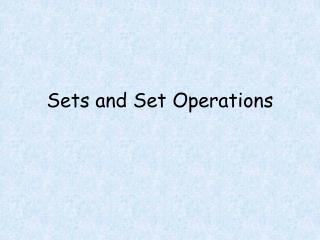DownloadDownload PresentationSets and Set Operations

Sets and Set Operations

Download PresentationSets and Set Operations

- - - - - - - - - - - - - - - - - - - - - - - - - - - E N D - - - - - - - - - - - - - - - - - - - - - - - - - - -
Presentation Transcript

1. Sets and Set Operations

2. Objectives • Determine if a set is well defined. • Write all the subsets of a given set and label the subsets as proper or improper. • Given a universal set and some subsets, find a complement, intersection or union. • Draw a Venn diagram to illustrate two sets. • Use the cardinal number formula.

3. Vocabulary • roster notation • set-builder notation • well defined set • cardinal number • empty set • subset • proper/improper subset • intersection of sets • union of sets • mutually exclusive • complement of a set

4. Set: roster notation: set builder notation: well defined set:

5. Determine if the given set is well defined. The set of all pink automobiles The set of all good bands The set of odd numbers The set of small numbers

6. Symbols related to sets:

7. Symbols related to sets:

8. Given the sets U = {0, 1, 2, 3, 4, 5, 6, 7, 8, 9},A = {0, 2, 4, 5, 6, 8}, andB = {1, 3, 5, 7}Answer the questions below: • Find n(B). • Find the set A B. • Find the set B . • Find the set A B.

9. Given the sets U = {0, 1, 2, 3, 4, 5, 6, 7, 8, 9},A = {0, 2, 4, 5, 6, 8}, andB = {1, 3, 5, 7}Answer the questions below: • Is 7A true or false? • Is 5B true or false?

10. Formulas Cardinal Number Formula for the Union of Sets n(AB) = n(A) + n(B) — n(AB) Cardinal Number Formula for the Complement of a Set n(U) = n(A) + n(A )

11. Suppose n(U) = 61, n(A) = 32, and n(B) = 26. If n(AB) = 40, find n(AB) and draw a Venn diagram to illustrate the composition of U.

12. In a recent health survey, 750 single men in their twenties were asked to check the appropriate box or boxes on the following form. I am a member of a private gym. I am a vegetarian. The results were tabulated as follows: 374 checked the gym box 92 checked the vegetarian box 332 were blank (no boxes checked)

13. 750 men surveyed 374 checked the gym box 92 checked the vegetarian box 332 were blank (no boxes checked) • Draw a Venn diagram illustration the results of the survey. • What percent of these men were both members of a private gym and vegetarians.

14. Cards

15. Cards Determine how many cards, in an ordinary deck of 52, are clubs or twos.

16. Cards Determine how many cards, in an ordinary deck of 52, are face cards or diamonds.

17. Cards Determine how many cards, in an ordinary deck of 52, are threes or sixes.

18. Cards Determine how many cards, in an ordinary deck of 52, are threes and sixes.